﻿ 一种基于熵权法的小波去噪复合评价指标文章快速检索 高级检索
 大地测量与地球动力学2018, Vol. 38Issue (7): 698-702,718  DOI: 10.14075/j.jgg.2018.07.008### 引用本文WANG Xu, WANG Chang. A Kind of Wavelet De-Noising Composite Evaluation Index Based on Entropy Method[J]. Journal of Geodesy and Geodynamics, 2018, 38(7): 698-702,718.### Foundation support

Research Projects of Liaoning Forestry Vocation-Technical College, No. KJ201505.

### 第一作者简介

WANG Xu, lecturer, majors in deformation monitoring and prediction, E-mail:wangxu19830411@126.com.

### 文章历史

1. 辽宁林业职业技术学院林学院，沈阳市枫杨路186号，110101;
2. 辽宁科技大学土木工程学院，辽宁省鞍山市千山中路185号，114051

1 传统评价指标

 $\begin{array}{l} M = 3\sin \left( {2{\mathit{p}_\mathit{t}}/500} \right) + 2\sin \left( {2{p_t}/200} \right) + \\ \;\;\;\;\;\;\;\;\;\;\;\;\sin \left( {2{p_t}/50} \right) + 0.001t \end{array}$ (1)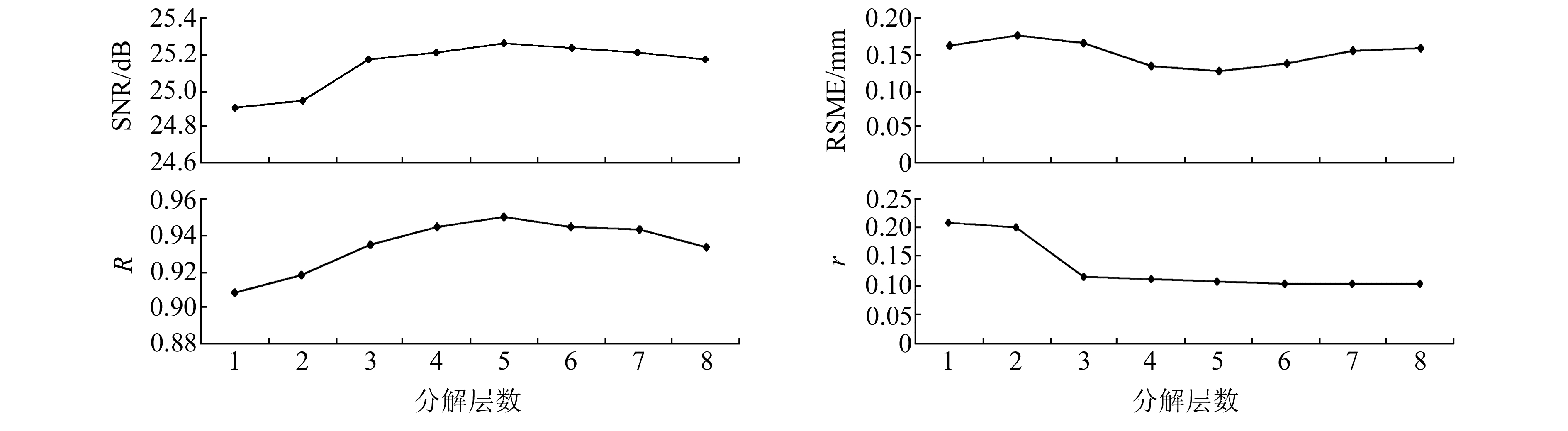图 1 4种传统评价指标对模拟变形数据M去噪效果的评价结果 Fig. 1 Evaluation results of the four traditional evaluation indexes on M simulation of deformation data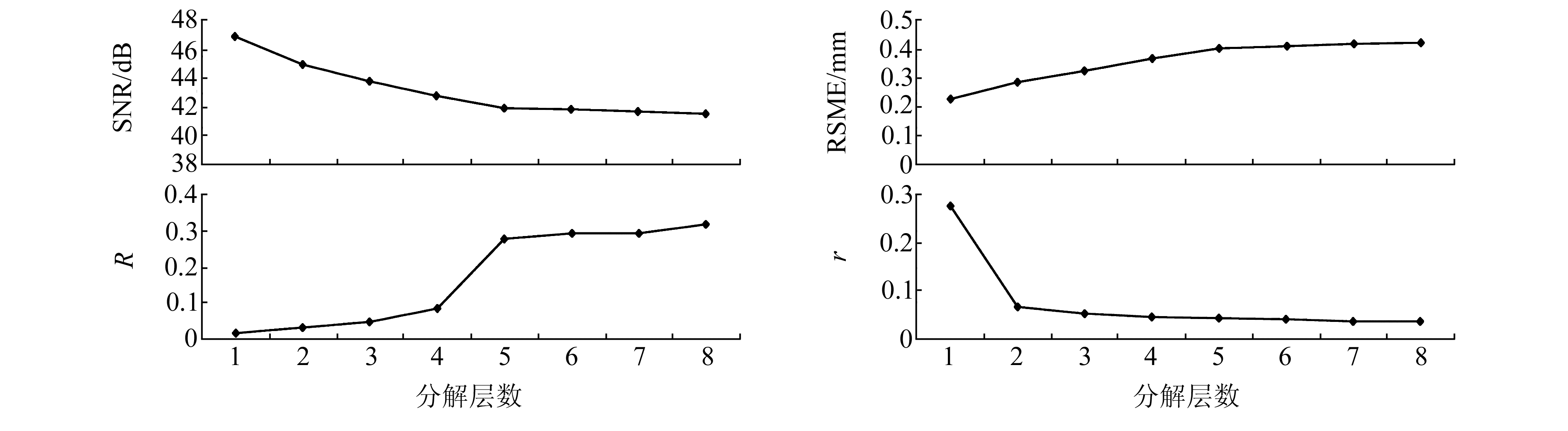图 2 4种传统评价指标对沉降信号去噪效果的评价结果 Fig. 2 Evaluation results of the four traditional evaluation indexes on settlement signals de-noising
2 构建新的评价指标

2.1 指标的选取

2.2 复合指标的构建

 ${P_{{\mathit{V}_\mathit{R}}}}\left( \mathit{k} \right) = \frac{{{\mathit{V}_{{\mathit{R}_{{\rm{max}}}}}} - {\mathit{V}_\mathit{R}}\left( \mathit{k} \right)}}{{{V_{{R_{\max }}}} - {V_{{R_{\min }}}}}}$ (2)

 ${P_{{\mathit{V}_\mathit{r}}}}\left( \mathit{k} \right) = \frac{{{\mathit{V}_\mathit{r}}\left( k \right) - {\mathit{V}_{{\mathit{r}_{{\rm{max}}}}}}}}{{{V_{{r_{\max }}}} - {V_{{r_{\min }}}}}}$ (3)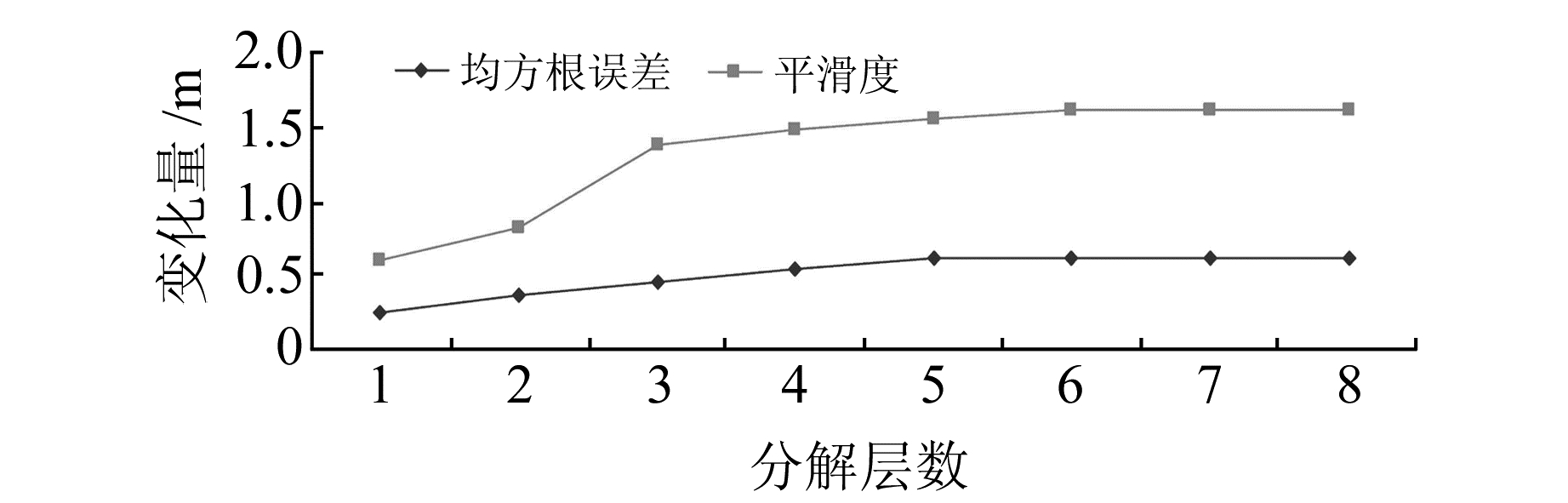图 3 均方根误差变化量以及平滑度变化量 Fig. 3 Root mean square error variation and smoothness variation

 $S={{w}_{{{P}_{{{V}_{R}}}}}}\left( k \right){{P}_{{{V}_{R}}}}\left( k \right)+{{w}_{{{P}_{{{V}_{r}}}}}}\left( k \right){{P}_{{{V}_{r}}}}\left( k \right)$ (4)

3 算例分析与比较表 1 不同分解层数的4种评价指标数据 Tab. 1 Four index data of the different decomposition level表 2 4种指标指导小波去噪后信号建立预测模型的精度等级 Tab. 2 Four indexes guide the wavelet de-noising signal to establish the accuracy grade of the prediction mode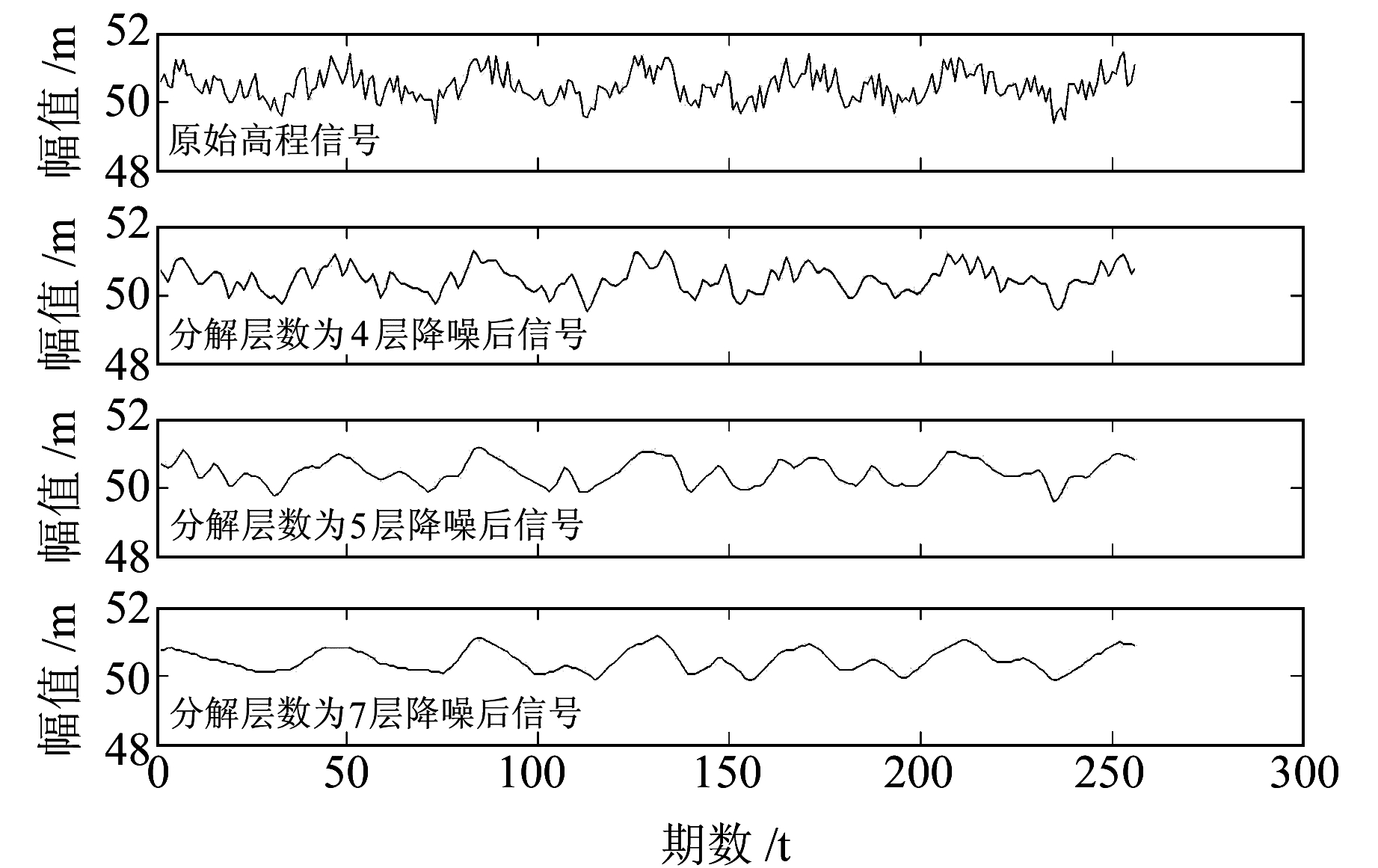图 4 不同分解层数的小波去噪效果图 Fig. 4 De-noising effect drawing of the different decomposition level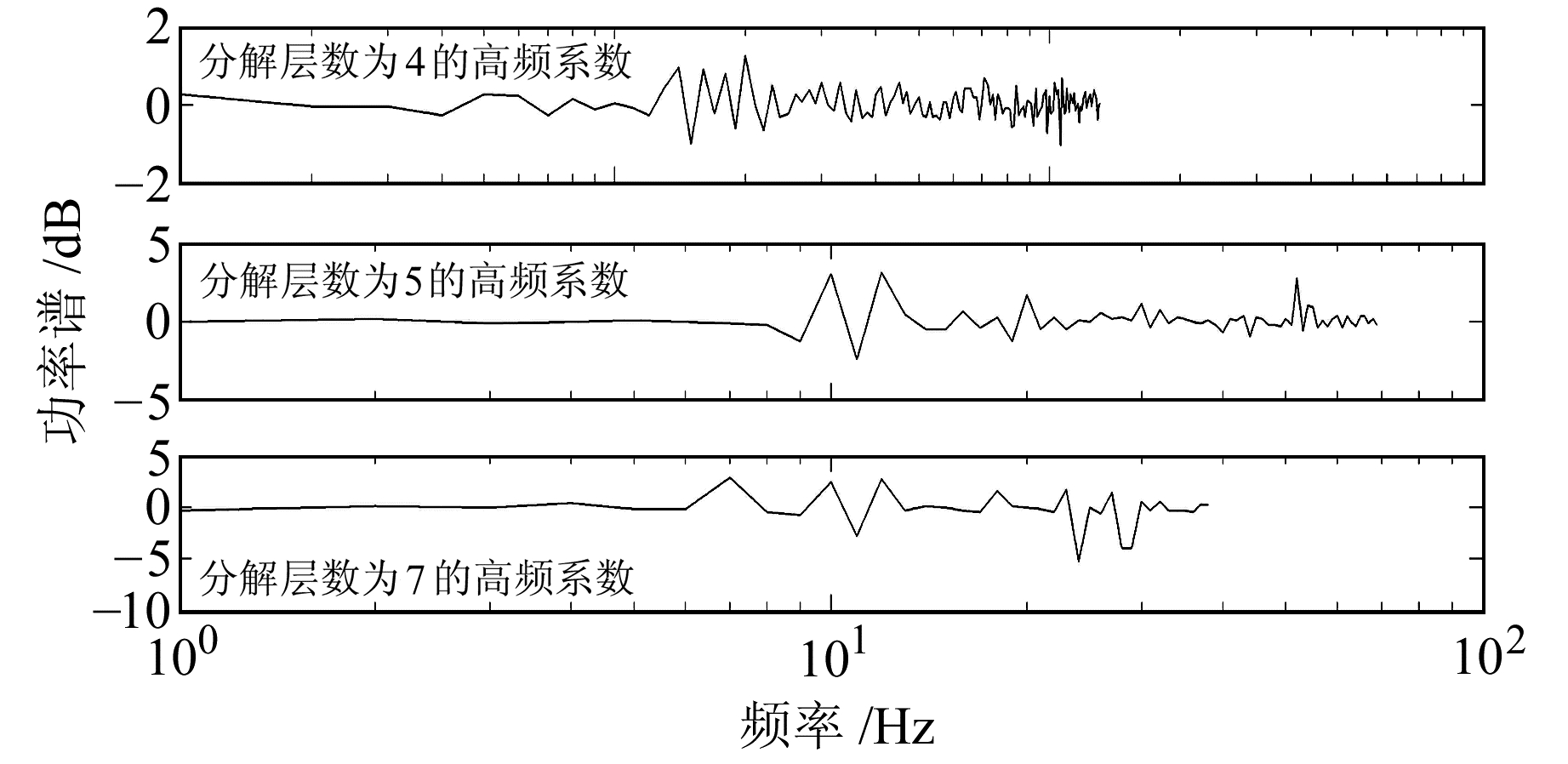图 5 功率谱密度 Fig. 5 Power spectrum density表 3 各分解层数的评价指标数据 Tab. 3 Evaluation indexes date of each decomposition level表 4 5层分解后的信号建立预测模型精度等级 Tab. 4 The signal of 5 layer decomposition establish accuracy grade of the prediction model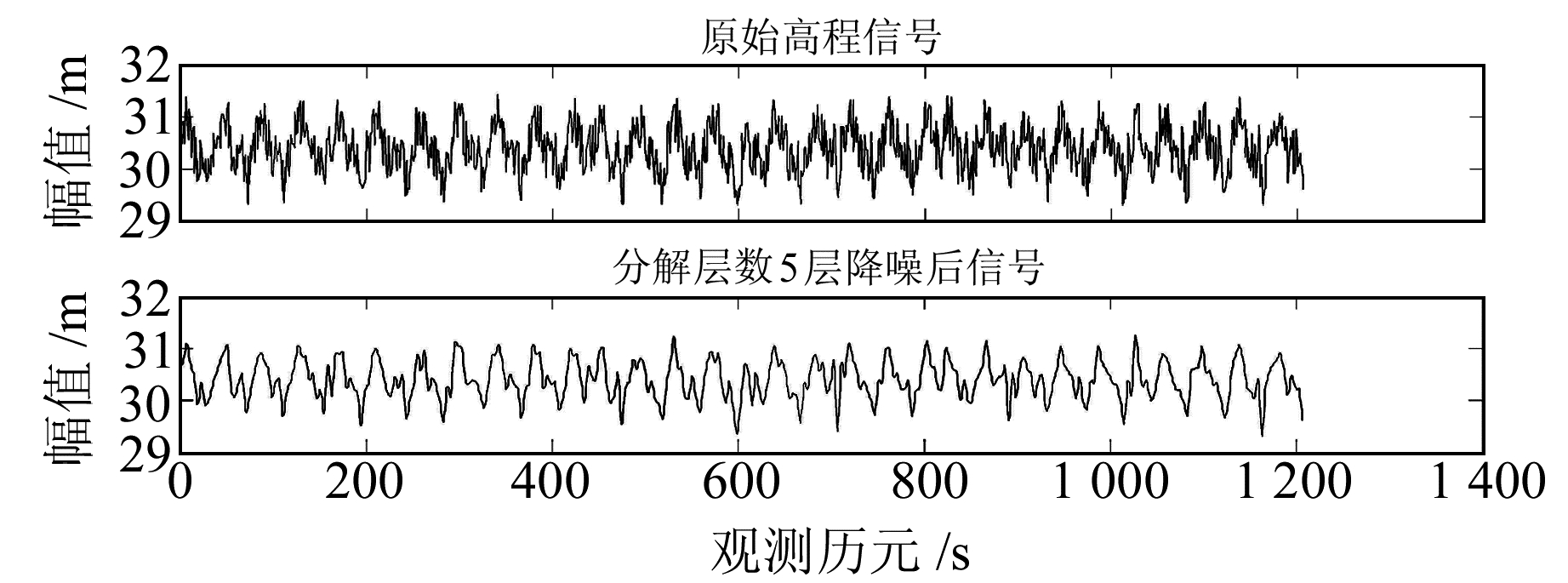图 6 分解层数为5的小波去噪效果 Fig. 6 De-noising effect drawing of five decomposition levels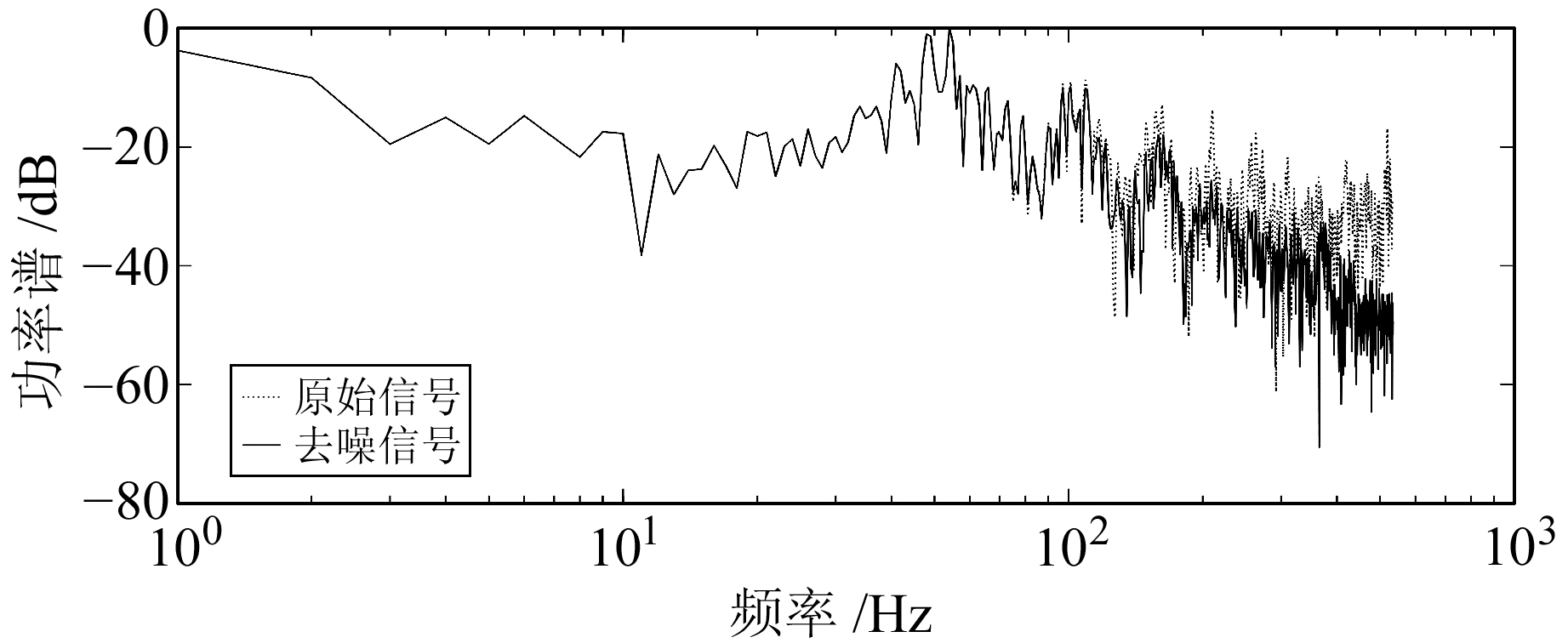图 7 功率谱密度 Fig. 7 Power spectrum density
4 结语

  王中元, 周天强, 张鹏飞. 高层建筑物GPS动态变形监测数据处理[J]. 测绘科学, 2012, 37(1): 47-50 (Wang Zhongyuan, Zhou Tianqiang, Zhang Pengfei. GPS Dynamic Deformation Monitoring on High-Rise Buildings[J]. Science of Surveying and Mapping, 2012, 37(1): 47-50) (0)  朱建军, 章浙涛, 匡翠林, 等. 一种可靠的小波去噪质量评价指标[J]. 武汉大学学报:信息科学版, 2015, 40(9): 688-693 (Zhu Jianjun, Zhang Zhetao, Kuang Cuilin, et al. A Reliable Evaluationg Indicator of Wavelet De-Noising[J]. Geomatics and Information Science of Wuhan University, 2015, 40(9): 688-693) (0)  郭健, 查吕应, 庞有超. 基于小波分析的深基坑地表沉降预测研究[J]. 岩土工程学报, 2014, 36(增2): 343-347 (Guo Jian, Zha Lüying, Pang Youchao. Prediction for Ground Settlement of Deep Excavations Based on Wavelet Analysis[J]. Hinese Journal of Geotechnical Engineering, University, 2014, 36(S2): 343-347) (0)  李宗春, 邓勇, 张冠宇, 等. 变形测量异常数据处理中小波变换最佳级数的确定[J]. 武汉大学学报:信息科学版, 2011, 36(3): 285-288 (Li Zongchun, Deng Yong, Zhang Guanyu, et al. Determination of Best Grading of Wavelet Transform in Deformation Measurement Data Filtering[J]. Geomatics and Information Science of Wuhan University, 2011, 36(3): 285-288) (0)  陶珂, 朱建军. 多指标融合的小波去噪最佳分解尺度选择方法[J]. 测绘学报, 2012, 41(5): 749-755 (Tao Ke, Zhu Jianjun. A Hybrid Indicator for Determining the Best Decomposition Scale of Wavelet Denoising[J]. Acta Geodaetica et Cartographica Sinica, 2012, 41(5): 749-755) (0)  黄其欢, 岳建平. 地基InSAR新技术及水利工程变形监测应用[J]. 辽宁工程技术大学学报, 2015, 34(3): 386-389 (Huang Qihuan, Yue Jianping. A New Technology of Ground-Based InSAR and Its Application to Hydraulic Engineering Deformation Monitoring[J]. Journal of Liaoning Technical University, 2015, 34(3): 386-389) (0)  Xu K J, Wang X F, Li Y S. Fundamental Wave Extraction and Frequency Measurement Based on IIR Wavelet Filter Banks[J]. Measurement, 2007, 40(6): 665-671 DOI:10.1016/j.measurement.2006.07.010 (0)  张德丰. Matlab小波分析[M]. 北京: 机械工业出版社, 2009 (Zhang Defeng. Matlab Wavelet Analysis[M]. Beijing: China Machine Press, 2009) (0)  吴奕, 朱海兵, 周志成. 基于熵权模糊物元和主元分析的变压器状态评价[J]. 电力系统保护与控制, 2015, 43(17): 1-6 (Wu Yi, Zhu Haibing, Zhou Zhicheng.. Transformer Condition Assessment Based on Entropy Fuzzy Matter-Element and Principal Component Analysis[J]. Power System Protection and Control, 2015, 43(17): 1-6 DOI:10.7667/j.issn.1674-3415.2015.17.001) (0)  罗毅, 李昱龙. 基于熵权法和灰色关联分析法的输电网规划方案综合决策[J]. 电网技术, 2013, 37(1): 77-80 (Luo Yi, Li Yulong. Comprehensive Decision-Making of Transmission Network Planning Based on Entropy Weight and Grey Relational Analysis[J]. Power System Technology, 2013, 37(1): 77-80) (0)  周伟萍, 王丰效. 灰色DNGM(1, 1)预测模型及其优化[J]. 计算机工程与应用, 2013, 49(10): 28-31 (Zhou Weiping, Wang Fengxiao. DNGM(1, 1)Model and Its Parameters Optimal[J]. Computer Engineering and Applications, 2013, 49(10): 28-31 DOI:10.3778/j.issn.1002-8331.1202-0322) (0)  王利, 张勤, 张显云. 基于均值滤波的灰色预测模型及其应用[J]. 西安科技大学学报, 2008, 28(1): 67-71 (Wang Li, Zhang Qin, Zhang Xianyun. Application of Object-Oriented Analysis Models in Expert System of Welding Process Planning[J]. Journal of Xi'an University of Science and Technology, 2008, 28(1): 67-71) (0)
A Kind of Wavelet De-Noising Composite Evaluation Index Based on Entropy Method
WANG Xu1WANG Chang21. Forestry Institute, Liaoning Forestry Vocation-Technical College, 186 Fengyang Road, Shenyang 110101, China;
2. College of Civil Engineering, University of Science and Technology Liaoning, 185 Mid-Qianshan Road, Anshan 114051, China
Abstract: The traditional evaluation index cannot meet the requirements of wavelet de-noising quality evaluation if the truth value is unknown. Using rate-change characteristics, this paper proposes a new evaluation index that reconstructs the root mean square error variation evaluation index and smoothness variation evaluation index. We use the normalized entropy weight method to linearly combine the two normalized evaluation indices. This new index is the composite evaluation index. The method decides the optimal de-noising layer by means of the obvious change of the rate of change of the index as the number of decomposition layers increases. Experimental data analysis shows that this method can guide wavelet decomposition accurately, and accurately determines the optimal de-noising layer to achieve the optimal de-noising effect when the truth value is unknown.
Key words: deformation monitoring; wavelet de-noising; evaluation index; entropy method; GM(1, 1) model